Today, I’ll try to give some insights about TDA (for Topological Data Analysis), a mathematical field quickly evolving, that will certainly soon be completely integrated into machine-/deep- learning frameworks. Some use-cases will be presented in the wake of this article, in order to illustrate the power of that theory !

### Quick History

Topological Data Analysis, also abbreviated TDA, is a recent field that emerged from various works in applied topology and computational geometry. It aims at providing well-founded mathematical, statistical and algorithmic methods to exploit the topological and underlying geometric structures in data. You will generally find it suitable for three-dimensional data, but experience shows that TDA reveals also to be useful in other cases, such as time-series.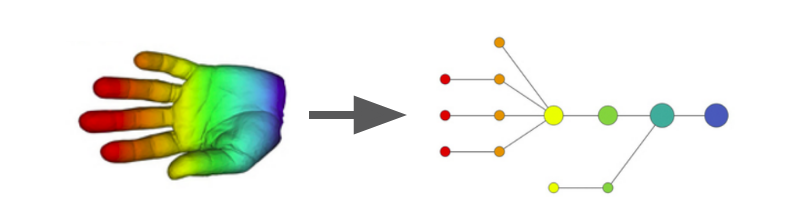Example of Mapper construction based on the height function

There are a variety of theories defined in topology, and its influence in machine-learning or deep-learning is constantly growing. Who ever interested in those out-of-the-box theories that are able to bring unexpected insights about data may be interested by what’s coming next ! However, concerning this article, I’ll focus on visual examples, and limit myself to one- and two-dimensional spaces for comprehension purposes.

### Persistence Homology

Among the most widespread theories, there is persistence homology. It is a method aimed at computing topological features of a space at different spatial resolutions. By construction, those features are more likely to represent true characteristics of the underlying space (rather than artifacts of sampling, noise, or particular choice of parameters) as they are intrinsically linked to the spatial relationships of the data points. To compute the persistence homology of a space, it must first be represented as a nested family of simplicial complex (basically a graph made of a set of points and their relationships aka lines and triangles in a two-dimensional space). This family is called a filtration. Generally, the construction of this filtration is based on the definition of a distance function, whose values are used to index the complexes in the family. The choice of this distance is thus of great importance, and gives food for thoughts for the ones interested in metric learning …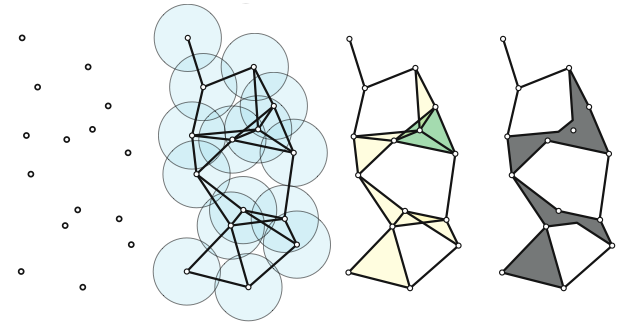Visual Construction of the Simplicial Complex

The theory of persistence homology allows us to uniquely represent the persistence homology of a filtered simplicial complex with a persistence barcode or persistence diagram. A barcode diagram represents each persistence generator with a horizontal line beginning at the first filtration level where it appears, and ending at the filtration level where it disappears, while a persistence diagram plots a point for each generator with its x-coordinate the birth time and its y-coordinate the death time (examples are given below). Visually, in two dimensions, you consider each point independently: You draw a circle of increasing radius around those points until you find some intersections (birth time). You pursue the drawing until you cover some points and destroy some of the previously created structures (death time). You finally end up with components (date of birth and death), which are represented through those persistence diagram and barcode.

### Persistence Diagrams

To better visualize this theory, let’s consider the example of a 1 dimensional time-serie, noted f. We want to focus on the critical points of f by the following rule: When a new component is introduced, we say that the local minimum that creates it represents the component. When we pass a local maximum and merge two components, we pair the maximum with the higher (younger) of the two local minima that represent the two components. The other minimum is now the representative of the component resulting from the merger. When x and y are paired by this method we define the persistence of the pair to be f(y) − f(x). Persistence is represented in the persistence diagram by mapping each pair to the point (f(x), f(y)) whose coordinates are the corresponding critical values, as shown here under.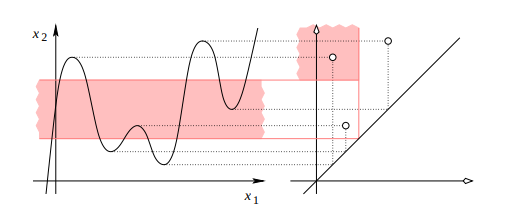Value-Based Persistence of a 1D Signal

### Vectorization and Representations

That’s great, but what to do next ?!? The issue of non-uniformity of the vectors extracted from persistence diagrams is a real drawback for machine-learning applications. To deal with it without necessarily looking deeper into new type of neural networks, we have to transform the previous persistence representations in something gathering somehow the same information, that may be sizable. For machine learning, extracting the outlying persistence points could represent great features, but some underlying information contained in the diagram could be lost through that process.

Here I’ll underline three possibilities: the Betti curves, the persistence landscapes and the persistence images. (The whole computation is made thanks to the Gudhi package, developed by the French team DataShape of the INRIA.) The following constructions are on their way to be scikit-learn compatibles. Meanwhile, I refer you to my corresponding Github repository for the persistence construction, and the diagrams’ representations. Let’s visualize this through the following example. Who ever dreamed of algorithmically counting the number of fingers you have on your hand will be pleased by what’s coming …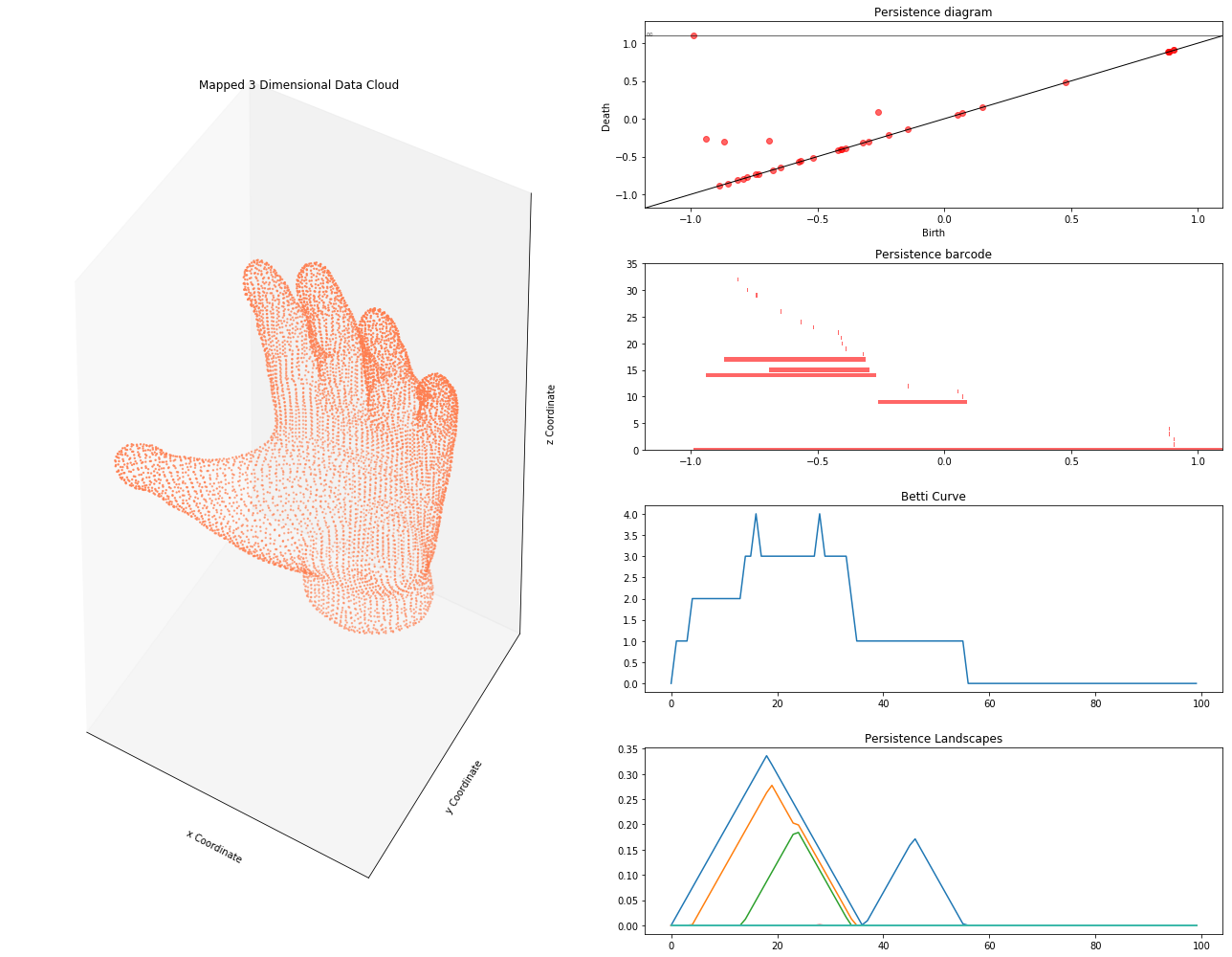TDA Representation of a 3D Object: Finger Characterization

What do we get from this result. First, you observe graphically through the persistence diagram and barcode that there are 5 highlighted components. Unsurprisingly, those are the 5 fingers ! However, one of the component is placed at the infinite: this corresponds to the first point encountered while considered the upper-levels of the filtration, automatically given an infinite value. As a consequence, your persistence landscapes only clearly represent the four smaller fingers. This is something to keep in mind while applying TDA !

Betti Curve: Let’s consider a persistence barcode, for which we vectorize the radius space. Each component of the barcode diagram is then referred to as a function taking 1 as value on the radius defining it and 0 anywhere else. The sum of those functions defines the Betti Curve.

Persistence Landscapes: Each component of the persistence diagram is referred to by a triangular function (slope of 1). The superposition of those triangular functions gives an apparent structure of mountains in different plans, of which we extract iteratively the upper landscapes.

Persistence Images: Each component of the persistence diagram becomes the center of a 2 dimensional gaussian distribution of a specific variance. By creating the corresponding matrix of distribution superposition, you obtain the image.

One should notice the following point: Even if the number of fingers appears clearly (corresponding to the points far from the diagonal), there remains a notion of topological noise (points close to the diagonal). As a consequence, the persistence landscapes are generally of better use since they remain more or less robust to it, as they preferably describe the most persistence objects of the dataset. Nonetheless, topological noise is sometimes useful information, typically when working with time-series whose standard deviation is of great importance for classification purposes …

### Deep-Learning Pipeline

Once you build, for all your samples, the corresponding persistence representations, you may wonder how to use it. The betti curves are kinda easy to feed into a 1D Convolutional network, while the persistence images are suitable for a 2D Convolutional network.

Concerning the persistence landscapes, it gets a bit trickier. For the ones that wonder how to fully exploit it without handcrafting your own features from it, here is a solution I built. Basically, it is linked to the idea of persistence silhouette, which is a weighted sum of the triangular functions obtained from the persistence diagram. The newly created layer corresponds to a weighted sum of those persistence landscapes, getting as output a moving-average structure of the persistence silhouette. The output is a 1-dimensional signal that you can feed into a 1D Convolutional network. I’ll further develop this idea in incoming work ! 🙂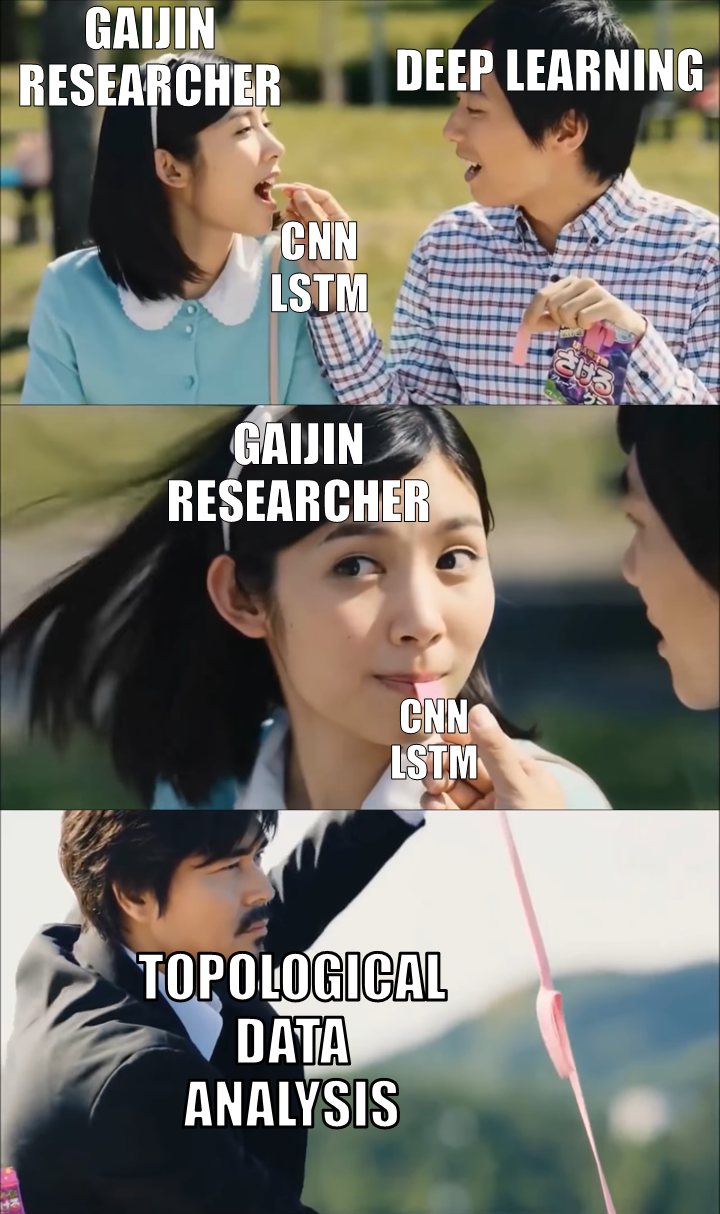Credits: Gaijin et al.

There are lots of possibilities emerging from this theory, and the interface between those results and deep-learning are currently still under development. Lots of ideas are to come, which make the topic hot and really interesting ! Stay tuned for the incoming articles, and do not forget to give you clap if you want more about it 😉 !

### References

Content retrieved from: https://towardsdatascience.com/from-tda-to-dl-d06f234f51d.